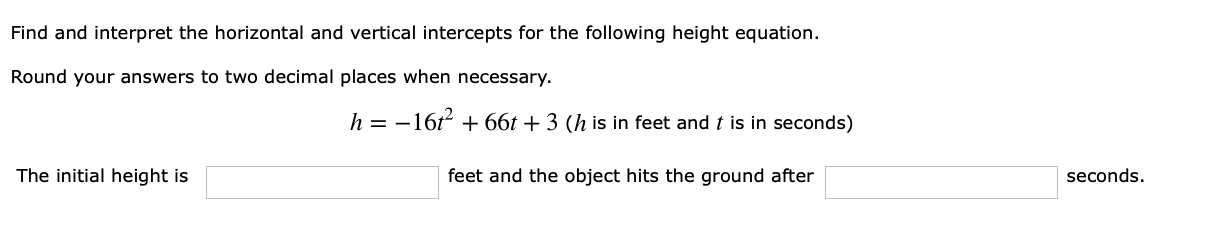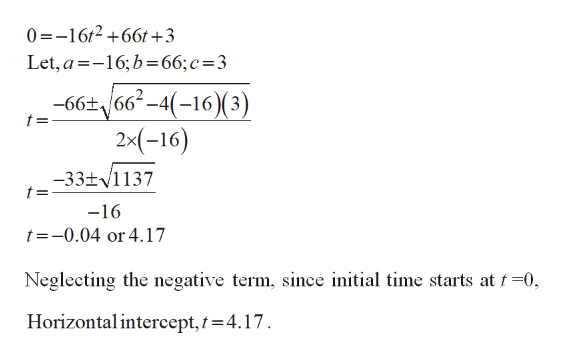# Find and interpret the horizontal and vertical intercepts for the following height equation.Round your answers to two decimal places when necessary.h=1666t + 3 (h is in feet and t is in seconds)The initial height isfeet and the object hits the ground afterseconds.

Question
5 viewshelp_outlineImage TranscriptioncloseFind and interpret the horizontal and vertical intercepts for the following height equation. Round your answers to two decimal places when necessary. h=16 66t + 3 (h is in feet and t is in seconds) The initial height is feet and the object hits the ground after seconds. fullscreen
check_circle

Step 1

Find the horizontal and vertical intercepts of the equation and also find initial height and the object hit the ground.

Step 2

For finding horizontal intercept,

Substi...help_outlineImage Transcriptionclose0162+66t+3 Let, a-16; b66; c=3 -66t66-4(-16)(3) 2x(-16) t= -33t1137 -16 t=-0.04 or 4.17 Neglecting the negative term, since initial time starts at t =0 Horizontalintercept, t 4.17 fullscreen

### Want to see the full answer?

See Solution

#### Want to see this answer and more?

Solutions are written by subject experts who are available 24/7. Questions are typically answered within 1 hour.*

See Solution
*Response times may vary by subject and question.
Tagged in

### Applications of Mathematics